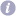»  Home »  Bookstore »  Title index »  Multidisciplinary »  Métodos Quantitativos com Stata

## Métodos Quantitativos com StataClick to enlarge
See the back cover

$15.50 Print$7.50 Discounted priceWhat are VitalSource eBooks?
Your access code will be emailed upon purchase.
eBook not available for this title

eBook not available for this title

 Authors: Luiz Paulo Fávero, Patrícia Belfíore, Renata Turola Takamatsu, and Janilson Suzart Publisher: Elsevier Copyright: 2014 ISBN-13: 978-85-352-5157-9 Pages: 248; paperback Price: $15.50$7.50
 Authors: Luiz Paulo Fávero, Patrícia Belfíore, Renata Turola Takamatsu, and Janilson Suzart Publisher: Elsevier Copyright: 2014 ISBN-13: Pages: 248; eBook Price: $0.00  Authors: Luiz Paulo Fávero, Patrícia Belfíore, Renata Turola Takamatsu, and Janilson Suzart Publisher: Elsevier Copyright: 2014 ISBN-13: Pages: 248; Kindle Price:$

### Comment from the Stata technical group

Métodos Quantitativos com Stata contains applied material that is appropriate for a diverse number of users in areas such as social science, biostatistics, and engineering. The book describes a combination of alternative resources to execute commands in Stata. It illustrates the interactive use and the menu-driven modes for the general examples included in all the chapters.

The first two chapters describe the basic elements for using Stata for data management and basic statistics. The authors show some of the commands for tables, graphs, and univariate and multivariate tests for normality. In chapter three, they use the ttest command to test for the equality of means in one and two samples, and they dedicate one whole section to the basic commands for the analysis of variance.

The next three chapters focus on linear regression. Chapter four presents basic applications for simple and multivariate regression analysis, and it includes some of the available tests for violations of the assumptions of the general linear model. There is also a simple time-series application, including a test for autocorrelation. Chapter five continues with the postestimation analysis by working with the commands for determining potential strategies in the presence of outliers. There is a discussion on the need to transform the variables to overcome problems that could be present in a regression because of heteroskedasticity, skewness, kurtosis, and scaling issues. In chapter six, the authors start by illustrating the predict options to detect outliers. They use a simple example to show the syntax and the menu steps to obtain the statistics for leverage, cook's distance, DFITS, and COVRATIO. They then use different alternatives to fit linear regression models in the presence of heteroskedasticity and outliers. They discuss the use of the "robust" and "cluster" options and the command for linear robust regressions (rreg). The chapter finishes with an example using quantile regression, which is less sensitive to outliers compared with OLS.

In chapter seven, the authors introduce the concepts associated with the logistic model and discuss the differences between fitting the model with OLS and using the logistic regression estimator. There is a good illustration of some of the available diagnostic tools to evaluate the regression results. They show the use of estat class to report the classification table and classification statistics, including specificity and sensitivity. They also work with the postestimation command lroc to evaluate the discriminatory power of the model. They finish this subsection presenting the results produced by the logistic command and provide the interpretation for the odds ratios in the logistic model. The chapter ends with the extension to multinomial logistic regression. The authors illustrate the mlogit command and interpret the coefficient estimates associated with the relative-risk ratio. They also use the test command to test a couple of linear hypotheses on the parameters, and they show the graphic interpretation of the effect of the covariates on the probability of a positive outcome.

The first part of chapter eight describes the kinds of situations that correspond to the framework of survival analysis. It includes the definitions for the survival and hazard functions and the basic elements for the proportional hazards models and the accelerated failure-time models for survival analysis. The concepts are intuitively introduced by working with a study on the effect of a particular treatment on 100 patients. Most of the chapter is devoted to illustrating how Stata implements the Kaplan–Meier estimator and the Cox regression. The authors follow a step by step analysis showing the results for the Kaplan-Meier estimator using the sts list command, along with the commands for graphs and tests associated with the corresponding survival estimates. They then fit the Cox model to illustrate the use of covariates in survival analysis. They also show the commands to produce the graphs for the predicted survival and hazard functions and to test the proportional hazard assumption. They finish the chapter by comparing the results from the two estimators.

The last chapter introduces panel-data linear regression. It first shows the commands for specifying the panel structure and for producing panel summary statistics. The chapter then shows an applied example with a model on external causes for mortality using an artificial dataset. The authors fit the model with a few different estimators (pooled OLS, between and within estimators, and random effects) and interprets and compares the parameter estimates across the alternative sets of results. They also use the hausman command to decide between the fixed-effects and random-effects estimators. The last section of the chapter extends the model to account for serial correlated errors using the Stata panel-data commands that are explicitly designed to implement that type of estimation.